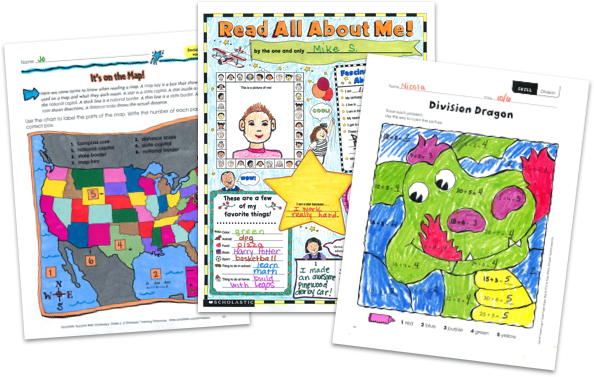MY FILE CABINET# Math for Kids From Scholastic TeachablesScholastic Teachables (formerly Scholastic Printables) has more than 6,000 "Math for Kids" tools and resources that span more than 20 different math skills! Sign up today and get instant online access to math worksheets, math lesson plans, practice pages, activities, games, and more to cover all grades and skill levels. Plus, you'll get access to Scholastic's more than 30,000 award-winning printables and leveled learning collections in reading and math to reach every learner.

Scholastic Teachables (formerly Scholastic Printables) has more than 6,000 "Math for Kids" tools and resources that span more than 20 different math skills! Sign up today and get instant online access to math worksheets, math lesson plans, practice pages, activities, games, and more to cover all grades and skill levels. Plus, you'll get access to Scholastic's more than 30,000 award-winning printables and leveled learning collections in reading and math to reach every learner.

## Our Math Printables Cover:

• Algebra
• Charts and Graphs
• Counting and Numbers
• Data Analysis
• Estimation
• Early Math
• Fractions and Decimals
• Geometry
• Glyphs
• Logic and Problem Solving
• Money
• Multiplication and Division
• Number Sense
• Order of Operations
• Perimeter, Area, Volume
• Probability
• Ratio, Proportion, Scale
• Real-World Math
• Sequencing
• Time and Measurement

## Sample Math for Kids Resources

More than 6,000 printable math resources and tools span more than 20 math topics and a wide range of skills from Pre-K through grade 8. Scholastic publishes new printable math worksheets each month to keep your courses fresh, exciting, and up-to-date, and you can access all of our resources from any device, whether at school or at home. Spend more time focusing on your courses and less time on preparing materials—simply find it, print it, teach it!

## Our Math Printables Cover:

• Algebra
• Charts and Graphs
• Counting and Numbers
• Data Analysis
• Estimation
• Early Math
• Fractions and Decimals
• Geometry
• Glyphs
• Logic and Problem Solving
• Money
• Multiplication and Division
• Number Sense
• Order of Operations
• Perimeter, Area, Volume
• Probability
• Ratio, Proportion, Scale
• Real-World Math
• Sequencing
• Time and Measurement
• Algebra
• Charts and Graphs
• Counting and Numbers
• Data Analysis
• Estimation
• Early Math
• Fractions and Decimals
• Geometry
• Glyphs
• Logic and Problem Solving
• Algebra
• Charts and Graphs
• Counting and Numbers
• Data Analysis
• Estimation
• Early Math
• Fractions and Decimals
• Geometry
• Glyphs
• Logic and Problem Solving
• Money
• Multiplication and Division
• Number Sense
• Order of Operations
• Perimeter, Area, Volume
• Probability
• Ratio, Proportion, Scale
• Real-World Math
• Sequencing
• Time and Measurement

## Sample Math for Kids Resources

More than 6,000 printable math resources and tools span more than 20 math topics and a wide range of skills from Pre-K through grade 8. Scholastic publishes new printable math worksheets each month to keep your courses fresh, exciting, and up-to-date, and you can access all of our resources from any device, whether at school or at home. Spend more time focusing on your courses and less time on preparing materials—simply find it, print it, teach it!## Additional Alphabet Worksheets (Subscription Required)

Try FREE for 30 days!

If you are not 100% satisfied cancel during the FREE trial and owe nothing. If you are not 100% satisfied cancel during the FREE trial and owe nothing.